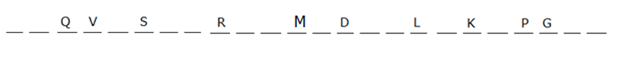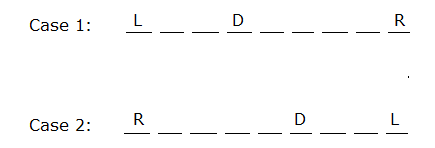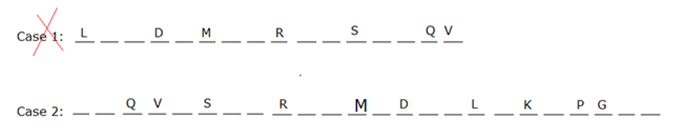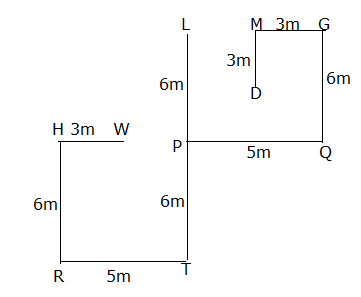# IBPS RRB Clerk Mains Reasoning Questions 2019 (Day-02)

Dear Aspirants, Our IBPS Guide team is providing new series of Reasoning Questions for IBPS RRB Clerk Mains 2019 so the aspirants can practice it on a daily basis. These questions are framed by our skilled experts after understanding your needs thoroughly. Aspirants can practice these new series questions daily to familiarize with the exact exam pattern and make your preparation effective.

Check here for IBPS RRB PO Mains Mock Test 2019

Check here for IBPS RRB Clerk Mains Mock Test 2019

Check here for IBPS PO Prelims Mock Test 2019

### Click Here to Subscribe Crack High Level Puzzles & Seating Arrangement Questions PDF 2019 Plan

[WpProQuiz 6938]

Directions (1-4): Study the following information to answer the given questions:

3412 8672 9537 3815 9714

1) If 1 is added to each even digit and 1 is subtracted from each odd digit, then what is the sum of 3rd digit of third highest number and 2nd digit of second lowest number?

a) 10

b) 12

c) 11

d) 15

e) None of these

2) If second and third digits are interchanged, 1st and 4th digits are interchanged in each number, then what will be the difference between second digit of highest number and fourth digit of second lowest number?

a) 7

b) 5

c) 4

d) 3

e) None of these

3) If 2 is added to each odd number and 2 is subtracted from each even number, then what is the product of fourth digit of highest number and second digit of second lowest number?

a) 15

b) 21

c) 24

d) 16

e) None of these

4) If all the numbers are arranged in descending order, then 1 is added to each number, then what is the sum of 4th digit of second number from left end and 3rd digit of second number from the right end?

a) 10

b) 9

c) 7

d) 5

e) None of these

Directions (5-7): Study the following information to answer the given questions:

Certain number of persons are sitting in a row facing north. Four persons sit between R and D. More than six persons sit between L and R. Only one person sits between M and D. S and R are not immediate neighbours. More than four persons sit between L and S. Two persons sit between L and D. Two persons sit between Q and S. L and M are not immediate neighbours. As many persons person sit between S and G is thrice as many persons sit between Q and R. Only two persons sit to the right of G. Less than thirteen persons sit between R and G. Five person sit between S and M. Two persons sit between K and G. V sits immediate right of Q. Only one person sits between P and K. As many persons sit to the right of P sit to the left of V.

5) How many persons are sitting in a row?

a) 25

b) 28

c) 34

d) 24

e) None of these

6) Which of the following is true?

a) Less than five persons sit between S and D

b) Three persons sit between Q and S

c) More than fourteen persons sit between K and V

d) As many persons sit to the left of S sits to the right of K

e) None is true

7) How many persons sit between L and V?

a) 10

b) 12

c) 11

d) 14

e) None of these

Directions (8-10): Study the following information to answer the given questions:

Distance between Point L and Point T is 12m. P is midpoint of Point L and Point T. Point L and Point T are on Y-axis. Point Q is 5m east of Point P. Distance between Point PL and Point GQ is same. Point G is north of Point Q. Point M is 3mwest of Point G. Point D is 3m south of Point M. Point R is 5m west of Point T. Point H is 6m north of Point R. Point W is 3m east of Point H. Point M is east of Point L.

8) If Point J is 3msouth of Point D, then how far and in which direction Point W with respect to Point J?

a) 5m, West

b) 4m, East

c) 5m, East

d) 4m, West

e) None of these

9) Point T is in which direction with respect to Point D?

a) North West

b) South West

c) South East

d) North East

e) None of these

10) Four of the following five are alike in a certain way based on the group. Find the one which does not belongs to the group?

a) GT

b) QR

c) LH

d) MP

e) LQ

Directions (1-4):

3412 8672 9537 3815 9714

2503 9763 8426 2904 8605

2+9=11

3412 8672 9537 3815 9714

2143 2768 7359 5183 4179

8-3=5

3412 8672 9537 3815 9714

3410 8670 9539 3817 9712

2*8=16

3412 8672 9537 3815 9714

9715 9538 8673 3816 3413

8+1=9

Directions (5-7):Four persons sit between R and D. Two persons sit between L and D. More than six persons sit between L and R.Only one person sits between M and D. Five person sits between S and M. S and R are not immediate neighbours. L and M are not immediate neighbours. More than four persons sit between L and S. Two persons sit between Q and S. V sits immediate right of Q. As many persons person sits between S and G is thrice as many persons sits between Q and R. Only two persons sit to the right of G. Less than thirteen persons sit between R and G. Two persons sit between K and G. Only one person sits between P and K. As many person sits to the right of P sits to the left of V.Case 1 will be dropped because less than thirteen persons sit between R and G.

Directions (8-10):